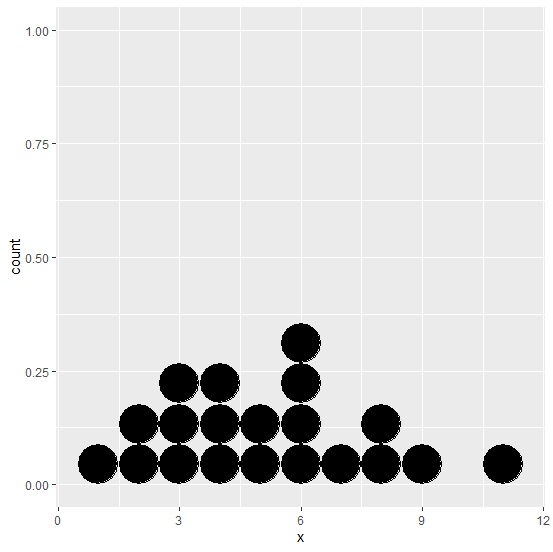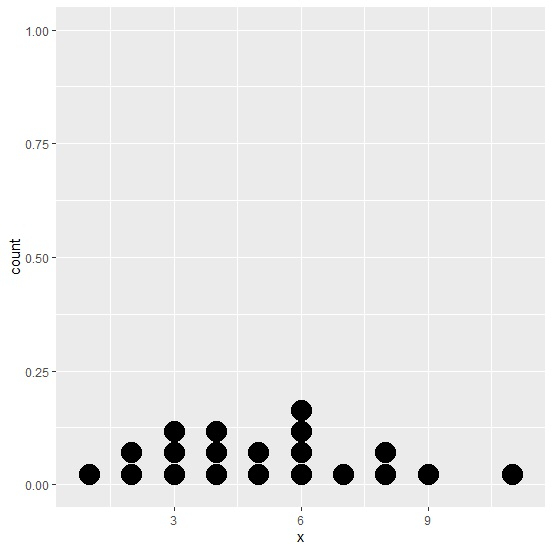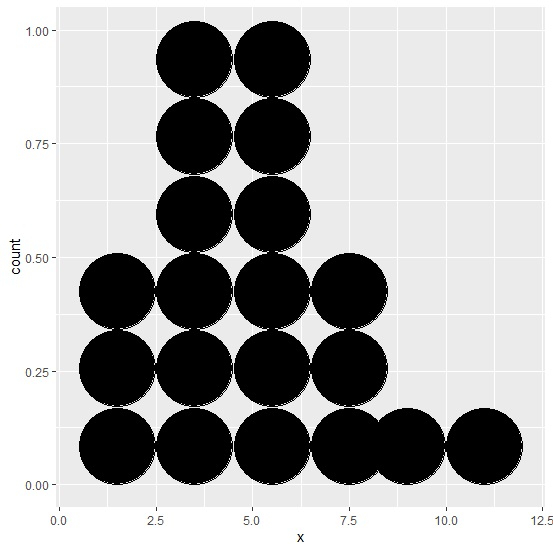# How to change the size of dots in dotplot created by using ggplot2 in R?

To change the size of dots in dotplot created by using ggplot2, we can use binwidth argument inside geom_dotplot. For example, if we have a data frame called df that contains a column x for which we want to create the dotplot then the plot with different size of dots can be created by using the command ggplot(df,aes(x))+geom_dotplot(binwidth=2).

## Example

Consider the below data frame −

Live Demo

x<-rpois(20,5)
df<-data.frame(x)
df

## Output

   x
1  1
2  3
3  6
4  3
5  5
6  11
7  2
8  3
9  2
10 6
11 5
12 4
13 4
14 6
15 8
16 6
17 8
18 9
19 4
20 7

Loading ggplot2 package and creating a dotpot for data in df with different size of dots −

## Example

library(ggplot2)
ggplot(df,aes(x))+geom_dotplot(binwidth=1)

## Output## Example

ggplot(df,aes(x))+geom_dotplot(binwidth=0.5)

## Output## Example

ggplot(df,aes(x))+geom_dotplot(binwidth=2)

## Output